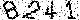Position: Home > Circuit Diagram > Basic Circuit > Use the OR circuit, composed of diodes and resistors C = A + B circuit diagram

# Use the OR circuit, composed of diodes and resistors C = A + B circuit diagramTo evaluate my
Code: